# KSEEB SSLC Class 10 Maths Solutions Chapter 9 Polynomials Ex 9.2

In this chapter, we provide KSEEB SSLC Class 10 Maths Solutions Solutions Chapter 9 Polynomials Ex 9.2 for English medium students, Which will very helpful for every student in their exams. Students can download the latest KSEEB SSLC Class 10 Maths Solutions Solutions Chapter 9 Polynomials Ex 9.2 pdf, free KSEEB SSLC Class 10 Maths Solutions Solutions Chapter 9 Polynomials Ex 9.2 pdf download. Now you will get step by step solution to each question.

### Karnataka State Syllabus Class 10 Maths SolutionsChapter 9 Polynomials Ex 9.2

Question 1.
Find the zeroes of the following quadratic polynomials and verify the relationship between the zeroes and their coefficients
(i) x2 – 2x – 8
(ii) 4s2 – 4s + 1
(iii) 6x2 – 3 – 7x
(iv) 4u2 – 8u
(v) t2 – 15
(vi) 3x2 – x – 4
Solution: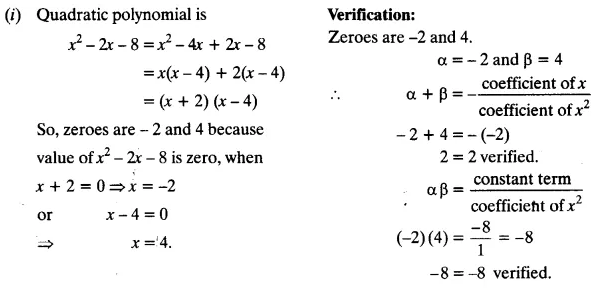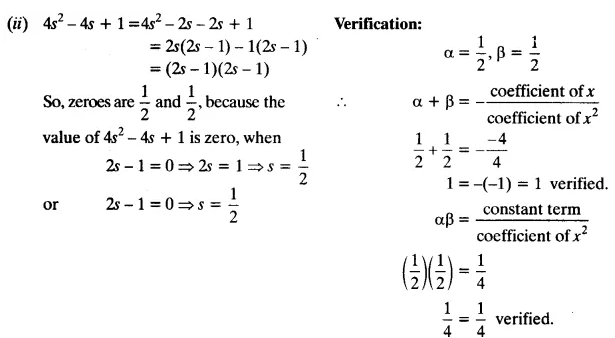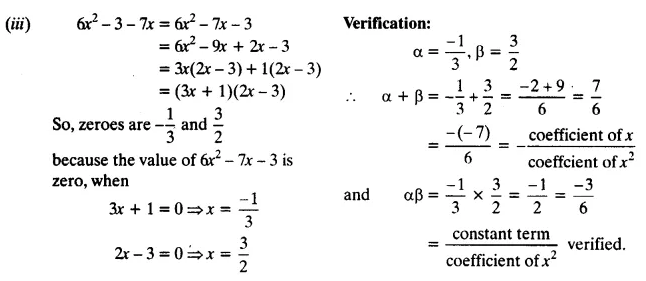(iv) 4u2 – 8u
= 4u2 – 8u + 0
= 4u (u – 2)
If 4u = 0, then u = 0
If u – 2 = 0, then u = 2
∴ Zeroes are 0 and 2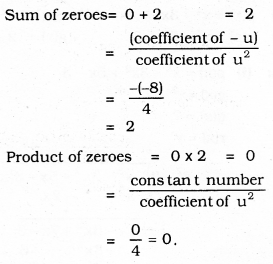(v) t2 – 15
= t2 + 0 – 15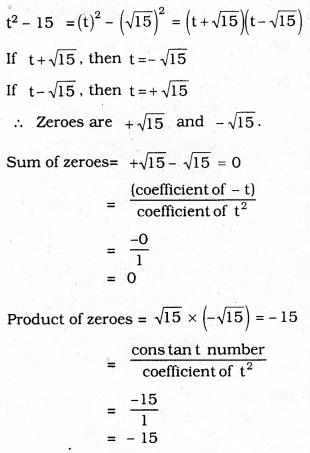(vi) 3x2 – x – 4
= 3x2 – 4x + 3x – 4
= x(3x – 4) + 1 (3x – 4)
= (3x – 4) (x + 1)
If 3x – 4 = 0, then x = 43
If x + 1 = 0, then x = -1
∴ Zeroes are 43 and -1.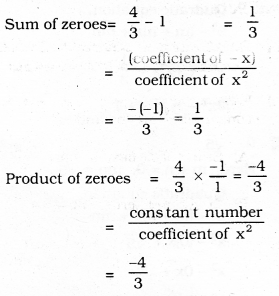Question 2.
Find a quadratic polynomial each with the given numbers as the sum and product of its zeroes respectively.
(i) 14, 1
(ii) 2–√,13
(iii) 0, 5–√
(iv) 1, 1
(v) −14,14
(vi) 4, 1
Solution:
(i) 14, 1
Here m + n = 14, mn = -1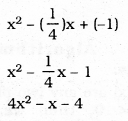(ii) 2–√,13
Here m + n = 2–√, mn = 13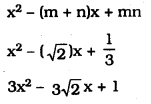(iii) 0, 5–√
Here m + n = 0, mn = 5–√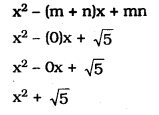(iv) Standard form of quadratic polynomial
sum and product of its zeroes is
K(x2 – sum of the zeroes) x + product of zeroes.
= K(x2 – 1x + 1)
Taking K = 1
= x2 – x + 1

(v) −14,14
Here m + n = −14, mn = 14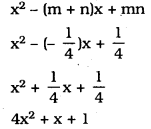(vi) Standard form of quadratic polynomial sum and product of its zeroes is
= K[x2 – (sum of the zeroes) x + Product of zeroes]
= K(x2 – 4x + 1)
Taking K = 1
= 1(x2 – 4x + 1)
= x2 – 4x + 1

All Chapter KSEEB Solutions For Class 10 Maths

—————————————————————————–

All Subject KSEEB Solutions For Class 10

*************************************************

I think you got complete solutions for this chapter. If You have any queries regarding this chapter, please comment on the below section our subject teacher will answer you. We tried our best to give complete solutions so you got good marks in your exam.

If these solutions have helped you, you can also share kseebsolutionsfor.com to your friends.

Best of Luck!!